# Colleges with the lowest SAT scores in Maine

Top 10 colleges in Maine with the lowest SAT scores
Looking for the colleges with the lowest SAT scores in Maine? Well you're in luck! We've compiled a national college database and have created a list of the top 10 universities with the lowest SAT scores in Maine below. If you are not a good test taker or worried about your test scores, this list is for you. These are the schools whose applicants had the lowest average SAT scores in Maine, which means that you can get into these colleges with a lower SAT score. We also include each college's ACT scores and acceptance rate so that you can see where you would have the easiest time getting in. Read on to find out more.

## University of Maine at Presque Isle SAT scores

The average SAT score for University of Maine at Presque Isle is 998.The average SAT score of 998 breaks down into:

• SAT math: 490

The average ACT score for University of Maine at Presque Isle is 20 and their acceptance rate is 91.6%.

## University of Maine Fort Kent SAT scores

The average SAT score for University of Maine Fort Kent is 1000.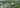The average SAT score of 1000 breaks down into:

• SAT math: 490

The average ACT score for University of Maine Fort Kent is 20 and their acceptance rate is 95%.

## Saint Joseph's College of Maine SAT scores

The average SAT score for Saint Joseph's College of Maine is 1040.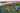The average SAT score of 1040 breaks down into:

• SAT math: 510

The average ACT score for Saint Joseph's College of Maine is 22 and their acceptance rate is 88.1%.

## Husson University SAT scores

The average SAT score for Husson University is 1060.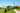The average SAT score of 1060 breaks down into:

• SAT math: 520

The average ACT score for Husson University is 20 and their acceptance rate is 80.2%.

## Unity College SAT scores

The average SAT score for Unity College is 1100.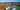The average SAT score of 1100 breaks down into:

• SAT math: 540

The average ACT score for Unity College is 22 and their acceptance rate is 88.7%.

## Maine Maritime Academy SAT scores

The average SAT score for Maine Maritime Academy is 1110.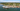The average SAT score of 1110 breaks down into:

• SAT math: 560

The average ACT score for Maine Maritime Academy is 22 and their acceptance rate is 78.6%.

## University of New England SAT scores

The average SAT score for University of New England is 1127.The average SAT score of 1127 breaks down into:

• SAT math: 560

The average ACT score for University of New England is 24 and their acceptance rate is 80.7%.

## University of Maine SAT scores

The average SAT score for University of Maine is 1154.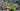The average SAT score of 1154 breaks down into:

• SAT math: 570

The average ACT score for University of Maine is 24 and their acceptance rate is 92.3%.

## College of the Atlantic SAT scores

The average SAT score for College of the Atlantic is 1269.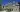The average SAT score of 1269 breaks down into:

• SAT math: 600

The average ACT score for College of the Atlantic is 28 and their acceptance rate is 67.9%.

## Bates College SAT scores

The average SAT score for Bates College is 1380.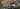The average SAT score of 1380 breaks down into:

• SAT math: 700